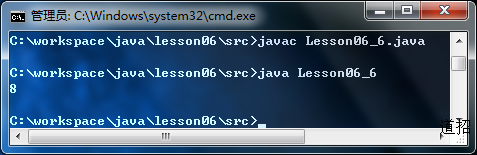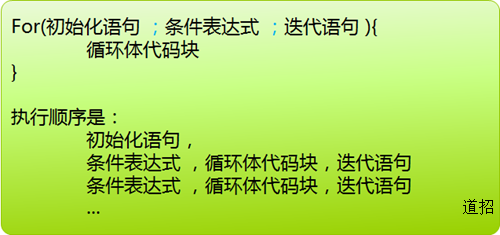# Java基础第六讲：流程控制（二）

#### Java基础第六讲：流程控制（二）

 `1` `public` `class` `Lesson06_6 {`
 `2` `    ``public` `static` `void` `main(String[] args) {`
 `3` `        ``int` `x = ``8``;`
 `4` `        ``while` `(x > ``0``) {`
 `5` `            ``System.out.println(x);`
 `6` `            ``x--;`
 `7` `        ``}`
 `8` `    ``}`
 `9` `}`

 `1` `public` `class` `Lesson06_6 {`
 `2` `    ``public` `static` `void` `main(String[] args) {`
 `3` `        ``int` `x = ``8``;`
 `4` `        ``do``{`
 `5` `            ``System.out.println(x);`
 `6` `            ``x--;`
 `7` `        ``}``while``(x>``8``);`
 `8` `    ``}`
 `9` `}`
x=8,条件是大于8，查看运行结果，我们发现他总是会执行一次。三、for 循环 当知道可以循环多少次时，是使用for循环的最佳时机。 1、基本for循环:先举一个例子：
 `1` `public` `class` `Lesson06_6 {`
 `2` `    ``public` `static` `void` `main(String[] args) {`
 `3` `        ``for` `(``int` `i = ``2``, j = ``1``; j < ``10``; j++) {`
 `4` `            ``if` `(j >= i) {`
 `5` `                ``System.out.println(i + ``"x"` `+ j + ``"="` `+ i * j);`
 `6` `            ``}`
 `7` `        ``}`
 `8` `    ``}`
 `9` `}`

1. for循环的三个部分任意部分都可以省略，最简单的for循环就是这样的 for(;;){ }
2. 中间的条件表达式必须返回一个布尔值，用来作为是否进行循环的判断依据
3. 初始化语句可以由初始化多个变量，多个变量之间可以用逗号隔开，这些在for循环中声明的变量作用范围就只在for循环内部
4. 最后的迭代语句可以是 i++,j++ 这样的表达式，也可以是毫无干系的 System.out.println(“哈哈”) 之类的语句，它照样在循环体执行完毕之后被执行。
2、for-each循环： for-each循环又叫增强型for循环，它用来遍历数组和集合中的元素，因此我们会在数组一章和集合一章里分别讲到，放心，你会掌握的很好。 这里举个例子给你看看先：
 `1` `public` `class` `Lesson06_6 {`
 `2` `    ``public` `static` `void` `main(String[] args) {`
 `3` `        ``int``[] a = { ``6``, ``2``, ``3``, ``8` `};`
 `4` `        ``for` `(``int` `n : a) {`
 `5` `            ``System.out.println(n);`
 `6` `        ``}`
 `7` `    ``}`
 `8` `}`

 `01` `public` `class` `BreakAndContinue {`
 `02`
 `03` `    ``public` `static` `void` `main(String[] args) {`
 `04`
 `05` `        ``int` `i =``0``;`
 `06` `        ``while``(``true``){`
 `07` `            ``System.out.println(``"i="``+i);`
 `08` `            ``if``(i==``12``){`
 `09` `                ``i++;`
 `10` `                ``i++;`
 `11` `                ``continue``;`
 `12` `            ``}`
 `13` `            ``i++;`
 `14` `            ``if``(i==``20``){`
 `15` `                ``break``;`
 `16` `            ``}`
 `17` `        ``}`
 `18`
 `19` `    ``}`
 `20`
 `21` `}`
 `22` `   `

 `01` `public` `class` `BreakAndContinue {`
 `02`
 `03` `    ``public` `static` `void` `main(String[] args) {`
 `04`
 `05` `        ``boolean` `isTrue = ``true``;`
 `06` `        ``outer:`
 `07` `            ``for``(``int` `i=``0``;i<``5``;i++){`
 `08` `                ``while``(isTrue){`
 `09` `                    ``System.out.println(``"Hello"``);`
 `10` `                    ``break` `outer;`
 `11` `                ``}`
 `12` `                ``System.out.println(``"Outer loop."``);`
 `13` `            ``}`
 `14` `        ``System.out.println(``"Good Bye!"``);`
 `15`
 `16` `    ``}`
 `17`
 `18` `}`
 `19` `   `

### 相关文章

#### Java基础第五讲：流程控制（一）

##### 关注道招网公众帐号##### 道招开发者二群